# 回顾transformer中绝对位置编码（absolute position embedding）

class BertEmbeddings(nn.Module):
def __init__(self, config):
super().__init__()
self.position_embeddings = nn.Embedding(config.max_position_embeddings, config.hidden_size) #初始化定义position_embedding的embedding层，其实底层就是一个fc
self.token_type_embeddings = nn.Embedding(config.type_vocab_size, config.hidden_size)
def forward(self, input_ids=None, token_type_ids=None, position_ids=None, inputs_embeds=None):
if input_ids is not None:
input_shape = input_ids.size()
else:
input_shape = inputs_embeds.size()[:-1]

seq_length = input_shape

if position_ids is None:
position_ids = self.position_ids[:, :seq_length] #生成定值的序列

if position_ids.dtype is not torch.long:
position_ids = position_ids.to(torch.long)

if token_type_ids is None:
token_type_ids = torch.zeros(input_shape, dtype=torch.long, device=self.position_ids.device)

if inputs_embeds is None:
inputs_embeds = self.word_embeddings(input_ids)
embeddings = inputs_embeds
embeddings += token_type_embeddings
if self.position_embedding_type == "absolute":
position_embeddings = self.position_embeddings(position_ids) #输入的固定序列经过一个fc得到位置编码，直接point-wise加回到token embedding上
embeddings += position_embeddings

embeddings = self.LayerNorm(embeddings)
embeddings = self.dropout(embeddings)
return embeddings



# 相对位置编码方法详解和公式推导

z i = ∑ j = 1 n α i j ( x j W V ) z_i = \sum^n_{j=1}\alpha_{ij}(x_jW^V)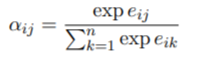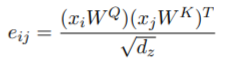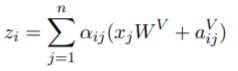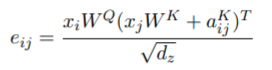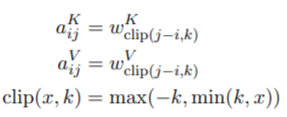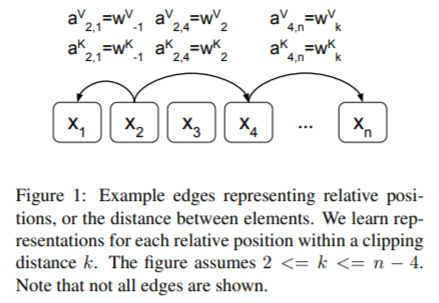# 相对位置编码代码实现

class BertSelfAttention(nn.Module):
def __init__(self, config):
super().__init__()
self.position_embedding_type = getattr(config, "position_embedding_type", "absolute")
if self.position_embedding_type == "relative_key" or self.position_embedding_type == "relative_key_query":
self.max_position_embeddings = config.max_position_embeddings
self.distance_embedding = nn.Embedding(2 * config.max_position_embeddings - 1, self.attention_head_size) #这里就是公式里的2k-1
def forward(
self,
hidden_states,
encoder_hidden_states=None,
output_attentions=False,
token_type_ids = None
):
mixed_query_layer = self.query(hidden_states)

# If this is instantiated as a cross-attention module, the keys
# and values come from an encoder; the attention mask needs to be
# such that the encoder's padding tokens are not attended to.
if encoder_hidden_states is not None:
mixed_key_layer = self.key(encoder_hidden_states)
mixed_value_layer = self.value(encoder_hidden_states)
else:
mixed_key_layer = self.key(hidden_states)
mixed_value_layer = self.value(hidden_states)

query_layer = self.transpose_for_scores(mixed_query_layer)
key_layer = self.transpose_for_scores(mixed_key_layer)
value_layer = self.transpose_for_scores(mixed_value_layer)

# Take the dot product between "query" and "key" to get the raw attention scores.
attention_scores = torch.matmul(query_layer, key_layer.transpose(-1, -2))

if self.position_embedding_type == "relative_key" or self.position_embedding_type == "relative_key_query":
seq_length = hidden_states.size()
position_ids_l = torch.arange(seq_length, dtype=torch.long, device=hidden_states.device).view(-1, 1)#生成[0,...,seq_length]的矩阵，生成两个相减就可以得到两两token之间的相对距离矩阵
position_ids_r = torch.arange(seq_length, dtype=torch.long, device=hidden_states.device).view(1, -1)
distance = position_ids_l - position_ids_r
positional_embedding = self.distance_embedding(distance + self.max_position_embeddings - 1) #都变成非负整数
positional_embedding = positional_embedding.to(dtype=query_layer.dtype)  # fp16 compatibility

if self.position_embedding_type == "relative_key":
relative_position_scores = torch.einsum("bhld,lrd->bhlr", query_layer, positional_embedding) #[贴一个einsum的详解](https://blog.csdn.net/a2806005024/article/details/96462827)
attention_scores = attention_scores + relative_position_scores
elif self.position_embedding_type == "relative_key_query":
relative_position_scores_query = torch.einsum("bhld,lrd->bhlr", query_layer, positional_embedding)
relative_position_scores_key = torch.einsum("bhrd,lrd->bhlr", key_layer, positional_embedding)
attention_scores = attention_scores + relative_position_scores_query + relative_position_scores_key